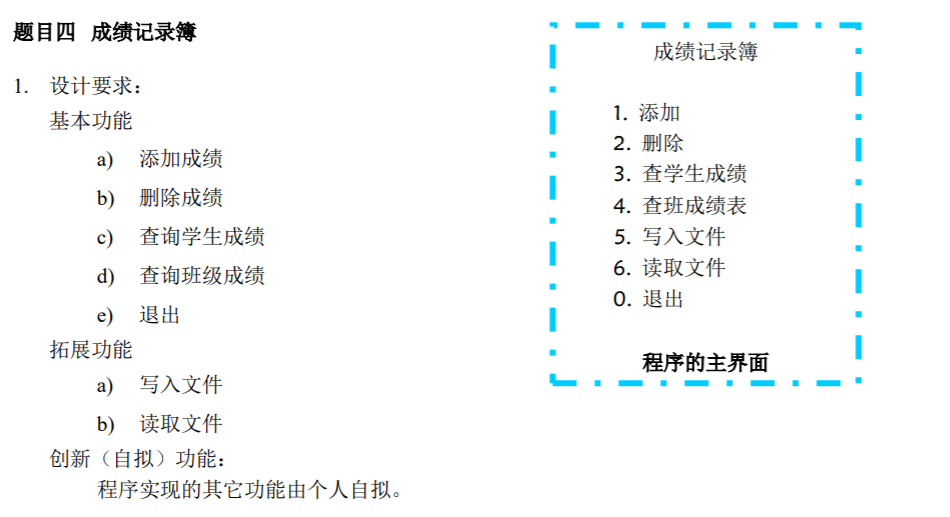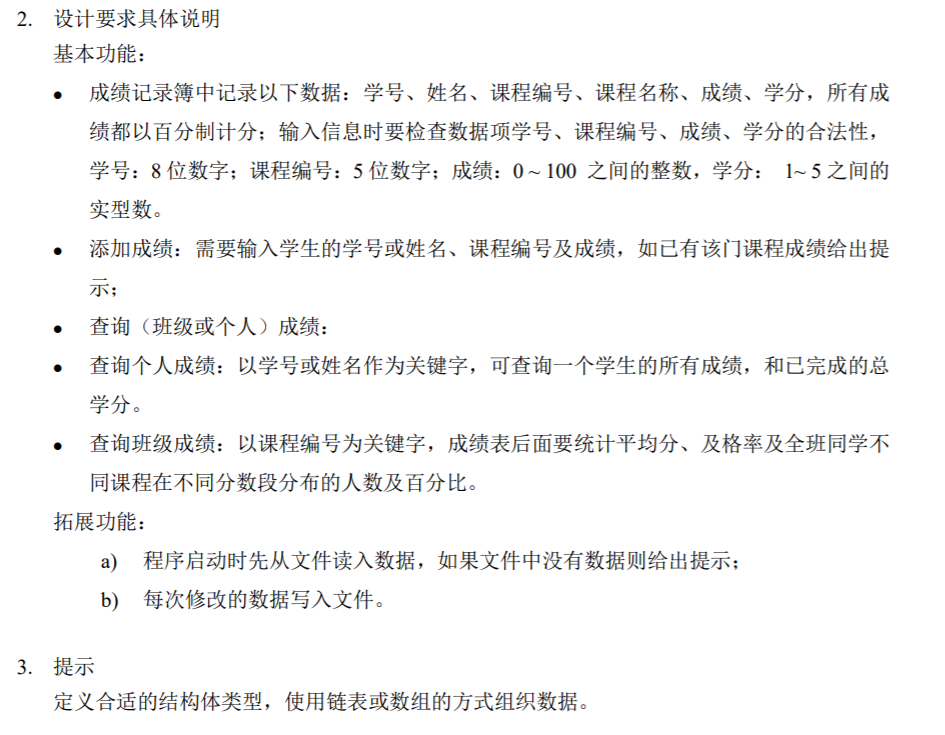• 很全面的，有源代码C++ 成绩记录课程设计，C++编程
• 《C语言程序设计学生成绩记录簿》由会员分享，可在线阅读，更多相关《C语言程序设计学生成绩记录簿(30页珍藏版)》请在人人文库网上搜索。1、课程实验报告课 程 名 称C语言程序设计13新能源2班实验日期2013年8月1号...

《C语言程序设计学生成绩记录簿》由会员分享，可在线阅读，更多相关《C语言程序设计学生成绩记录簿(30页珍藏版)》请在人人文库网上搜索。
1、课程实验报告课 程 名 称C语言程序设计13新能源2班实验日期2013年8月1号姓名许鑫学号6实验成绩实验学生成绩记录簿系统 名实验题目描述本程序是非数值计算型算法设计，我设计出了学生成绩记录簿系 统的基本功能，并设计了简单的界面。本程序主要考察对自定义函数 的熟悉程度，本程序主要使用的是链表的相关操作，包括结构体链表 的创建、输入、输出、查找、删除等。我设计的这个成绩记录簿系统 也初步实现了学生成绩信息的显示、输入、删除、查找等必要的功能。1. 建立一个主界面2. 用switch语句进行分类讨论3. 分別进入录入，查询，删除，等子函数。4. 将子函数与主函数相连即可。该程序可以建立学生的成绩。
2、记录。可以方便老师和同学方便的查阅成绩；这个程序有录入和删除的功能；还可以求出平均成绩，并排序成绩记录簿中记录以下数据：学号、姓名、课程编号、课程名称、 成绩、学分?添加成绩：需要输入学生的学号或姓名、课程编号及成绩，如 已有该门课程成绩给出提示；?查询(班级或个人)成绩：?查询个人成绩：以学号或姓名作为关键字，可查询一个学生的 所有成绩，和已完成的总学分。?查询班级成绩：以课程编号为关键字，成绩表后面要统计平均 分、及格率及全班同学不同课程在不同分数段分布的人数及百 分比。拓展功能：a)程序启动时先从文件读入数据，如果文件中没有数据则给 出提示；b)每次修改的数据写入文件实验环境以自己的电脑。
3、为主要的实验平台，运用Visual C+这个软件进行反 复的编写实验。1开始时2、添加半:3、査询4、査询成绩，E:匚运言字习Debugtest5e:e 丄 *1 -X1:1、添加；1：2、删除!；3、查学生成绩i*4、查班成绩裘!1 ； 5、写入文本文件1:6、读取文本文件1rr-.：0s退岀:I 选择操作：4黔入课廳号牆称1课程成绩课程学分|1110412?评鑫C语言1004.0000001平均分：1MW.UMUWUM及格率:丨半：-5写文本登找完毕车继续欢理使用学生,宓8记录鐘系统一- i 1、添加!1 2、删除!；3、直学生成绩；!仏奁班成绩表：!5、写人文本文件!16、读取文本文件I。
4、!队退出；选择操作：5请输入写入的文件地址；地址输入格式：例如d：Student.txt； |半：6、读取!3、査学生成绩!；4、查班成绩表；:5、写入文本文件I；6、读取文本文件；!a.退岀!算法描述及实验步骤选择操作：请输入读取的文件地址：地址输入格式,例如 dXstudont .txt ； o : Wat udon t .txt 读取中半:主程序提供一个界面，允许用户选择。使用switch语句，用时直 接输入数字即可。增加函数，功能是插入学生成绩。使用一个无穷循环，直到录入” n”时结束。删除函数，功能是删除，分为两步，先找后删。找，用strcmp函 数 来做如果找到记录i值，反之记为-。
5、1;删除可以从i项开始将数 组的后一项赋值给前一项。前一项自动被删掉。这样完成删除。查询函数与之类似，将删除改为输出即可；求平均值函数与查询类似只是输出时只输出平均值而已。按平均值排序只要加一个排序函数即可。退出函数什么都不写，即可。程序流程图调试 过 程实验 结果总结经过一个多星期的编译原理课程设计，在我的努力下，顺利完成 该课程设计。通过该课程设计，掌握了编译程序工作的基本过程及其各阶段的 基本任务，熟悉了编译程序总流程框图，了解了编译程序的生成过程 及其相关的技术，对课本上的知识也有了更深的理解。老师常说，课 本上的知识是机械的，表面的，要学会去运用，要举一反三。现在终 于深刻的了解了这。
6、句话。经过这次的课程设计，发现书本上很深奥的 知识变的更为简单，同样，对实验原理也了有更深的理解。知道和理 解了该理论在计算机中是怎样执行的，对该理论在实践中的应用有深 刻的理解。通过该课程设计，把死板的课本知识变得生动有趣，激发 了学习的积极性。能够把课堂上学的知识通过自己设计的程序表示出 来，加深了对理论知识的理解。课程设计中程序比较复杂，在调试时应该仔细，在程序调试时，注意 指针，将不必要的命令去除。除了课堂外，课程设计是最能学到东西 的，最考验人的。在做课程设计的这段时间，时刻都感到自己学的知 识有多么的贫瘠。经过这次课程设计，让我对C+这门语言有了更深 的认识，操作能力有了提高要想是。
7、写出的代码能运行，需要耐心细心， 毅力以及充沛的体力。只有经过多次编辑，多次编译，再多次运行，才能编写出更好的程序，有时候需要多次的更正才能达到所要的运行 结果。附录ftinclude ttinclude = MAX)return 0;for (i = StudentCount; i 100)printfC输入错误，请重新输入L)；return 0;printf (*ntt 课程学分：”)；scanf(%f, &Si Lj LessonCredit);i.f (Si. Lj. LessonCredit5)printf (”输入错误，请重新输入! ”)；:return 0;if (Si. Lj.。
8、 LessonMark StudentCount) printf (n未找到该学生nn*); return 0;elseStudentCount; return 1;void Student_Select()int x;char y100;char a100;int i, j；printfCn输入所査学生信息(1.姓名 2.学号)选择：”)； scanf (%d, &x);if (x = 1 | x = 2)if (x = 1)printf (*n 姓名：”)；fflush(stdin);gets(a);for (i = 0; i Si. Lj. LessonNumber,Si Lj. Les。
9、sonName,Si Lj, LessonMark,Si. Lj. LessonCredit);printf (”-n0 ;printf(nn);elseprintf (*n 学号：”)；fflush(stdin);gets(y);for (i = 0; i = 60)y+；break;printf (*n 平均分：ft 及格率：%f%nn*, z/x, y/x*100); printfCn査找完毕.回车继续n);get char ();void WriteF订e()FILE *fp;int i, j；char a100;printf Cn请输入写入的文件地址：nn地址输入格式：例如 d： ”。
10、)；scanf&a);printf (n 写入中n);if (fp = fopen (a,)fprintf (fp, *%dn*, StudentCount);for (i = 0; i StudentConnt; i+)fprintf (fp, %st%st%dn, Si. Number,S i. Name, S i LessonCount);for (j = 0; j SiLessonCount; j+) fprintf(fp, %st%st%dt%fn,Si. Lj. LessonNumber, Si. Lj. LessonName,Sil. Lj LessonMark, Si Lj LessonCredit);printf (*n写入完成.回车继续n);fclose (fp);elseprintfCn写入失败.回车继续n);get char ();void ReadFile()printf(n读取成功.回车继续n); fclose (fp);elseprintf(n读取失败.回车继续n);get char (。

展开全文• 成绩 成绩记录簿 C语言 C程序 记录簿 C语言 C程序 成绩记录簿 C语言 C程序
• 学生成绩记录簿设计 编制一个C语言成绩记录簿，每个学生信息包括：学号、姓名、C语言成绩。具体功能： （1）创建学生信息并以磁盘文件保存； （2）读取磁盘文件并显示输出所有学生的成绩； （3）按学号或姓名查询...JAVA 课程设计
• C语言程序设计报告学生成绩记录簿C语言程序设计报告-学生成绩记录簿上海应用技术学院课程设计2011 ~ 2012学年第 2 学期设计题目 成绩记录簿院(系) 计算机科学与信息工程 专 业 网络工程 班 级 学号 1110430115学生...

C语言程序设计报告学生成绩记录簿
C语言程序设计报告-学生成绩记录簿
上海应用技术学院
课程设计
2011 ~ 2012学年第 2 学期
设计题目 成绩记录簿
院(系) 计算机科学与信息工程 专 业 网络工程 班 级 学号 1110430115
学生姓名 xx
设计时间 2012.06.25~2012.07.06 指导教师 xxx
提交日期 2012.07.06
目录
1. 概述
1.1 题目
1.2程序简述
1.3程序性能
2 课题分析
2.1 程序系统的结构
2.2 结构流程图
2.3 功能
2.4 性能
2.5 输入输出项信息
3 功能模块说明
3.1 流程逻辑
3.2 算法
4 程序测试，运行结果及说明
5课程设计总结
6附录(参考文献，原代码解析，限制条件，程序测试)
6．1 参考文献
6．2 原代码解析
6.3限制条件
1.概述
1.1 题目
成绩记录簿
1.2程序描述
本程序是非数值计算型算法设计，我设计出了通讯录管理系统的基本功能，并设计了简单的界面。本程序主要考察对自定义函数的熟悉程度，本程序主要使用的是链表的相关操作，包括结构体链表的创建、输入、输出、查找、删除等。我设计的这个通讯录系统也初步实现了人员信息的显示、删除、查找、修改、添加等必要的功能。
1.建立一个主界面
2.用switch语句进行分类讨论
3.分别进入录入，查询，删除，求平均值等子函数。
4.将子函数与主函数相连即可。
1.3程序性能
该程序可以建立学生的成绩记录。可以方便老师和同学方便的查阅成绩；
这个程序有录入和删除的功能；
还可以求出平均成绩，并排序
2 课题分析
2.1程序系统的结构
2.2程序流程图
课程编号
2.3功能
成绩记录簿中记录以下数据：学号、姓名、课程编号、课程名称、成绩、学分，所有成绩都以百分制计分；输入信息时要检查数据项学号、课程编号、成绩、学分的合法性，学号：8位数字；课程编号：5位数字；成绩：0 ~ 100 之间的整数，学分： 1~ 5之间的实型数。
??添加成绩：需要输入学生的学号或姓名、课程编号及成绩，如已有该门课程成绩给出提示；
??查询(班级或个人)成绩：
??查询个人成绩：以学号或姓名作为关键字，可查询一个学生的所有成绩，和已完成的总学分。
??查询班级成绩：以课程编号为关键字，成绩表后面要统计平均分、及格率及全班同学不同课程在不同分数段分布的人数及百分比。
拓展功能：
a) 程序启动时先从文件读入数据，如果文件中没有数据则给出提示；
b) 每次修改的数据写入文件
2.4性能
方便,灵活，直观，易用
2.5 输入输出项信息
输入项：
char name 设置学生名称
long int id 定义学号为长整型
float avg 平均分为实数
输出项
int m1,m2,m3,score,xf; 定义整型
float avg; 以浮点型输出平均分
3 功能模块说明
3.1....................................................................... 流程逻辑
主程序提供一个界面，允许用户选择。使用switch语句，用时直接输入数字即可。
增加函数，功能是插入学生成绩。使用一个无穷循环，直到录入”n”时结束。
删除函数，功能是删除，分为两步，先找后删。找，用strcmp函数 来做如果找到记录i值，反之记为-1；删除可以从i项开始将数组的后一项赋值给前一项。前一项自动被删掉。这样完成删除。
查询函数与之类似，将删除改为输出即可；
求平均值函数与查询类似只是输出时只输出平均值而已。 按平均值排序只要加一个排序函数即可。 退出函数什么都不写，即可。
3．2 算法
本程序参考了冒泡法 其代码结构为： For(i=0;i
{
For(j=i;j
{
If(a>b)
{
}
}
}
其中也多次用到了循环和判断结构
3 运行结果及说明
1．开始时
2．添加
3．删除
4．查询学生成绩
(1)按名字查询
(2)按学号查询
4．查询班级成绩
5．写入文
6．读取文
4 课程设计总结
短短的俩周已经过去了，在此我将交出我一周的成果，我真是百感交错
1．原先写程序直接编，条理性太差。如果先做一个流程图就条理很多。
2．学

展开全文• 课程实验报告 课 程 13 新能源 2 实验日期 2013 年 8 月 C 语言程序设计 班级 名 班 1 号 称 姓 实验成绩 许鑫 学号 130370236 名 实 验 学生成绩记录簿系统 名 称 本程序是非数值计算型算法设计 我设计出了学生成绩...
• 课程实验报告 课 程 13 新能源 2 实验日期 2013 年8 月 C 语言程序设计 班级 名 班 1 号 称 姓 实验成绩 许鑫 学号130370236 名 实 验 学生成绩记录簿系统 名 称 本程序是非数值计算型算法设计 我设计出了学生成绩...
• 通过编写c语言《学生成绩记录簿系统》让我知道所学的编程语言是需要多练多编写代码锻炼出来的。 1）C语言课程设计的主要目的是培养学生综合运用C语言程序设计课程所学到的知识，编写C程序解决实际问题的能力，以及...
• 程序为用C写的成绩记录簿能帮助解决和提供程序设计的一些思路。
• 通过编写c语言《学生成绩记录簿系统》让我知道所学的编程语言是需要多练多编写代码锻炼出来的。 1）C语言课程设计的主要目的是培养学生综合运用C语言程序设计课程所学到的知识，编写C程序解决实际问题的能力，以及...
• 学生成绩记录簿 C语言课程设计 附：任务书，程序说明书、代码
• 学生成绩记录簿设计 编制一个C语言成绩记录簿,每个学生信息包括:学号、姓名、C语言成绩。具体功能 (1)创建信息链表并以磁盘文件保存; 2)读取磁盘文件并显示输出所有学生的成绩; (3)按学号或姓名查询成绩; 4)添加成绩...有问必答 c++ c语言
• 编制一个Java 语言成绩记录簿，每个学生信息包括：学号、姓名、Java语言成绩。具体功能： （1）创建学生信息并以磁盘文件保存； （2）读取磁盘文件并显示输出所有学生的成绩； （3）按学号或姓名查询成绩； （4）...java
• 1需求分析研究背景教育信息化必将涉及许多具体的技术和机器在教育中的应用，它使得一些人往往以技术论...而成绩管理系统将大大的提高老师的管理效率，使得老师可以针对学生的具体情况进行深入教育，培养跨世纪的创新...

1需求分析
研究背景
教育信息化必将涉及许多具体的技术和机器在教育中的应用，它使得一些人往往以技术论，机器论的观点来认识教育信息化的目的，认为教育信息化就是以机器代替教师讲课，代替教育写板书，就是以电子教材代替印刷教材，就是实现网上学习……，并以代替性、省力性、顺利性、效率性作为评价教育信息化的尺度。而成绩管理系统将大大的提高老师的管理效率，使得老师可以针对学生的具体情况进行深入教育，培养跨世纪的创新人才，是实现教育的现代化。教育信息化应以新的教育思想、教育观念指导信息技术在教育的各个部门、各个领域广泛应用，应根据创新人才培养的要求，利用信息技术，探索新的教育模式，促进教育现代化。只有在这样的基础上指导信息技术的教育应用，才是我们所需要的教育信息化。也就相应产生了对学生成绩的高效管理。
课题研究的目的及意义
现在我国各院高校的学生成绩管理水平普遍不高，有的还停留在纸质基础上，这种管理手段已不能适应时代的发展，因为它浪费了

展开全文• ## 程序设计基础实训 成绩记录簿

千次阅读 多人点赞 2019-09-10 19:18:51
程序设计基础实训 成绩记录簿 题目要求： C++代码如下： //2018-2019学年第二学期 程序设计基础实训（C++） //课程设计：成绩记录簿 /*预设student.dat： 【学号 姓名[张三]】 课程编号 科目...
程序设计基础实训 成绩记录簿
题目要求：C++代码如下：
//2018-2019学年第二学期 程序设计基础实训（C++）
//课程设计：成绩记录簿

/*预设student.dat：

【学号 姓名[张三]】
课程编号 科目[语文] 成绩 学分
课程编号 科目[数学] 成绩 学分
课程编号 科目[英语] 成绩 学分

【学号 姓名[李四]】
课程编号 科目[语文] 成绩 学分
课程编号 科目[数学] 成绩 学分
课程编号 科目[英语] 成绩 学分

【学号 姓名[王五]】
课程编号 科目[语文] 成绩 学分
课程编号 科目[数学] 成绩 学分
课程编号 科目[英语] 成绩 学分

*/

#include<iostream>
#include<iomanip>
#include<string>
#include<fstream>
using namespace std;

struct student
{
long number;
char name;
};

struct course
{
int course_number;
char course_name;
int score;
float credit;
};

struct data
{

student students;
int total_course;
course courses;
};

data s,s2;
int total_student=0;

{
int a,b,i,j,k,k2=1,m,v,p=0,choose=0,u=0,w,q,r;
cout<<"请输入即将输入的学生人数：";
do//判定人数是否超出数组可承受范围
{
cin>>a;
if(a>(100-total_student)) cout<<"超出最大限额！请重新输入：";
else k2=0;
}while(k2==1);
for(i=0;i<a;i++)
{
cout<<"请输入学号（8位数字）：";
do//判定学号是否为8位数字
{
cin>>s[i+total_student].students.number;
if((s[i+total_student].students.number<100000000)&&(s[i+total_student].students.number>9999999)) k=1;
else
{
k=0;
cout<<"格式有误！请重新输入：";
}
}while(k==0);
p=i+total_student;
for(v=0;v<total_student;v++)//判定该学生是否已记录在册
{
if(s[i+total_student].students.number==s[v].students.number)
{
p=v;
total_student--;
cout<<"当前学生："<<s[p].students.name<<endl;
goto leap1;
break;
}
}
cout<<"请输入学生的姓名：";
cin>>s[p].students.name;
leap1:
cout<<"请输入即将输入的学科个数：";
do//判定科目数量是否超出数组可承受范围
{
cin>>b;
k=0;
if(b>(10-s[p].total_course)) cout<<"科目数量超出最大限额！请重新输入：";
else k=1;
}while(k==0);
for(m=0;m<b;m++)
{
leap3:
cout<<"请输入课程编号（5位数字）：";
do//判定课程编号是否为5位数字
{
cin>>s[p].courses[m+s[p].total_course].course_number;
if(s[p].courses[m+s[p].total_course].course_number>100000||s[p].courses[m+s[p].total_course].course_number<9999)
{
k=1;
cout<<"格式有误！请重新输入：";
}
else k=0;
}while(k==1);
u=m+s[p].total_course;
for(q=0;q<m+s[p].total_course;q++)//判定该学生此课程编号是否已存在
{
if(s[p].courses[m+s[p].total_course].course_number==s[p].courses[q].course_number)
{
cout<<"该学生已有该科成绩，是否覆盖？"<<endl<<"1.覆盖成绩。"<<endl<<"2.重新输入课程编号。" <<endl;
leap4:
cin>>choose;
if(choose==1)
{
u=q;
s[p].total_course--;
goto leap2;
}
else if(choose==2)  goto leap3;
else
{
cout<<"请输入1或2进行选择：";
goto leap4;
}
}
}
for(w=0;w<i+total_student;w++)//判定所有数据中此课程编号是否已存在
{
for(r=0;r<s[w].total_course;r++)
{
if(s[p].courses[m+s[p].total_course].course_number==s[w].courses[r].course_number)
{
strcpy(s[p].courses[m+s[p].total_course].course_name,s[w].courses[r].course_name);
cout<<"当前课程："<<s[w].courses[r].course_name<<endl;
goto leap2;
}
}
}
cout<<"请输入课程名称：";
cin>>s[p].courses[u].course_name;
leap2:
cout<<"请输入成绩：";
do//判定成绩是否为0-100的整数
{
cin>>s[p].courses[u].score;
if(s[p].courses[u].score>100||s[p].courses[u].score<0)
{
k=0;
cout<<"请输入0-100的整数：";
}
else k=1;
}while(k==0);
cout<<"请输入学分：";
k=0;
do//判定学分是否为1-5的实型数
{
cin>>s[p].courses[u].credit;
if(s[p].courses[u].credit>5||s[p].courses[u].credit<1)
{
k=0;
cout<<"请输入1-5的实型数：";
}
else k=1;
}while(k==0);
}
s[p].total_course=s[p].total_course+b;
}
total_student=total_student+a;
}

void del()//删除成绩
{
int a,b,i,j,k,m,l,stu_number;
cout<<"请输入即将删除成绩的学生人数：";
do
{
cin>>a;
if(a>0&&a<=total_student) k=1;
else
{
k=0;
cout<<"超出目前学生人数！请重新输入：";
}
}while(k==0);
for(i=0;i<a;i++)
{
do
{
cout<<"请输入学号（8位数字）：";
cin>>s2[i].students.number;
for(j=0;j<100;j++)
{
if(s2[i].students.number==s[j].students.number)
{
stu_number=j;
k=1;
break;
}
else k=0;
}
if(k==0) cout<<"无此学生信息！请重新输入。"<<endl;
}while(k==0);
cout<<"请输入即将删除的学科个数：";
do
{
cin>>b;
if(b>0&&b<s[stu_number].total_course) k=1;
else
{
k=0;
cout<<"超出该学生目前已添加学科数目！请重新输入：";
}
}while(k==0);
for(m=0;m<b;m++)
{
do
{
cout<<"请输入课程编号（5位数字）：";
cin>>s2[stu_number].courses[m].course_number;
for(j=0;j<100;j++)
{
if(s2[stu_number].courses[m].course_number==s[stu_number].courses[j].course_number)
{
for(l=j;l<s[stu_number].total_course;l++)
s[stu_number].courses[l]=s[stu_number].courses[l+1];
k=1;
cout<<"删除成功！"<<endl;
break;
}
else k=0;
}
if(k==0) cout<<"无此成绩信息！请重新输入。"<<endl;
}while(k==0);
s[stu_number].total_course--;
}
}

}

void check_student()//查询学生成绩
{
int check_way,check_number,total_credit=0,i,j,k=0;
char check_name;
cout<<"1.学号查询"<<endl;
cout<<"2.姓名查询"<<endl;
leap7:
cin>>check_way;
if(check_way==1)
{
do
{
cout<<"请输入想要查询的学号：";
cin>>check_number;
for(i=0;i<total_student;i++)
{
if(check_number==s[i].students.number)
{
cout<<endl;
cout<<"学号："<<s[i].students.number<<endl;
cout<<"姓名："<<s[i].students.name<<endl;
cout<<"课程编号"<<setw(10)<<"课程名称"<<setw(8)<<"成绩"<<endl;
for(j=0;j<s[i].total_course;j++)
{
cout<<s[i].courses[j].course_number<<setw(10)<<s[i].courses[j].course_name<<setw(10)<<s[i].courses[j].score<<endl;
total_credit=total_credit+s[i].courses[j].credit;
}
cout<<"总学分："<<total_credit<<endl<<endl;
k=1;
break;
}
}
if(k==0) cout<<"无此学生信息！请重新输入。"<<endl;
}while(k==0);
}
else if(check_way==2)
{
do
{
cout<<"请输入想要查询的姓名：";
cin>>check_name;
for(i=0;i<100;i++)
{
if(strcmp(check_name,s[i].students.name)==0)
{
cout<<endl;
cout<<"学号："<<s[i].students.number<<endl;
cout<<"姓名："<<s[i].students.name<<endl;
cout<<"课程编号"<<setw(10)<<"课程名称"<<setw(8)<<"成绩"<<endl;
for(j=0;j<s[i].total_course;j++)
{
cout<<s[i].courses[j].course_number<<setw(10)<<s[i].courses[j].course_name<<setw(10)<<s[i].courses[j].score<<endl;
total_credit=total_credit+s[i].courses[j].credit;
}
cout<<"总学分："<<total_credit<<endl<<endl;
k=1;
break;
}
}
if(k==0) cout<<"无此学生信息！请重新输入。"<<endl;
}while(k==0);
}
else
{
cout<<"请输入1或2进行选择：";
goto leap7;
}
}

void check_class()//查询课程成绩
{
int check_coursenumber,i,j,l=0;
float sum=0,total_student=0,amount10090=0,amount8980=0,amount7970=0,amount6960=0,amount59=0;
float avg,avg_pass,avg90,avg80,avg70,avg60,avg59;
cout<<"请输入课程编号：";
leap6:
cin>>check_coursenumber;
for(i=0;i<100;i++)
{
for(j=0;j<s[i].total_course;j++)
{
if(check_coursenumber==s[i].courses[j].course_number)
{
l=1;
cout<<endl<<"课程名称："<<s[i].courses[j].course_name<<endl<<endl;
goto leap5;
}
}
}
if(l==0)
{
cout<<"无此课程信息！请重新输入：";
goto leap6;
}
leap5:
cout<<"学号"<<setw(14)<<"姓名"<<setw(10)<<"成绩"<<endl;
for(i=0;i<100;i++)
{
for(j=0;j<s[i].total_course;j++)
{
if(check_coursenumber==s[i].courses[j].course_number)
{
cout<<s[i].students.number<<setw(10)<<s[i].students.name<<setw(10)<<s[i].courses[j].score<<endl;
sum=sum+s[i].courses[j].score;
total_student++;
if(s[i].courses[j].score>=90) amount10090+=1;
else if(s[i].courses[j].score>=80&&s[i].courses[j].score<90) amount8980+=1;
else if(s[i].courses[j].score>=70&&s[i].courses[j].score<80) amount7970+=1;
else if(s[i].courses[j].score>=60&&s[i].courses[j].score<70) amount6960+=1;
else amount59+=1;
break;
}
}
}
avg=sum/total_student;
avg_pass=(amount10090+amount8980+amount7970+amount6960)*100/total_student;
avg90=amount10090*100/total_student;
avg80=amount8980*100/total_student;
avg70=amount7970*100/total_student;
avg60=amount6960*100/total_student;
avg59=amount59*100/total_student;
cout<<endl<<setiosflags(ios::fixed)<<setprecision(1)<<"平均分："<<avg<<endl;
cout<<"及格率："<<avg_pass<<"%"<<endl;
cout<<"分数100-90有"<<amount10090<<"人，占比"<<avg90<<"%"<<endl;
cout<<"分数89-80有"<<amount8980<<"人，占比"<<avg80<<"%"<<endl;
cout<<"分数79-70有"<<amount7970<<"人，占比"<<avg70<<"%"<<endl;
cout<<"分数69-60有"<<amount6960<<"人，占比"<<avg60<<"%"<<endl;
cout<<"分数小于60有"<<amount59<<"人，占比"<<avg59<<"%"<<endl<<endl;
}

void write_in(int &total_student)//写入文件
{
ofstream outfile("student.dat",ios::binary);
if(!outfile) cerr<<"文件写入错误，请重试！"<<endl;
else
{
outfile.write(reinterpret_cast<char *>(&total_student),sizeof(total_student));
for(int i=0;i<100;i++)
outfile.write((char*)&s[i],sizeof(s[i]));
outfile.close();
cout<<"文件写入成功！当前有"<<total_student<<"位学生的成绩数据。"<<endl<<endl;
}
}

void write_out(int &total_student)//读取文件
{
ifstream infile("student.dat",ios::binary);
if(!infile) cerr<<"文件读取错误，请重试！"<<endl;
else
{
for(int i=0;i<100;i++)
infile.close();
cout<<"文件读取成功！当前有"<<total_student<<"位学生的成绩数据。"<<endl<<endl;
}
}

int main()
{
int choose;
for(int i=0;i<100;i++)
{
s[i].total_course=0;
s2[i].total_course=0;
}

cout<<"自动读取文件……"<<endl;
write_out(total_student);

while(1)
{
cout<<"        成绩记录簿"<<endl<<endl;
cout<<"        1.添加"<<endl;
cout<<"        2.删除"<<endl;
cout<<"        3.查学生成绩"<<endl;
cout<<"        4.查班成绩表"<<endl;
cout<<"        5.写入文件"<<endl;
cout<<"        6.读取文件"<<endl;
cout<<"        0.退出"<<endl<<endl;
cout<<"请输入数字选择：";
cin>>choose;
switch(choose)
{
case 2:del();break;
case 3:check_student();break;
case 4:check_class();break;
case 5:write_in(total_student);break;
case 6:write_out(total_student);break;
case 0:exit(0);
default:;
}
}
return 0;
}


有很大缺陷，最直观的是学号是用的整型数字。 仅作留档。
展开全文• 2021年，自己写的，包含ppt答辩文稿，实验报告，源代码，容易看懂，不懂可以问我
• 用c++制作一个成绩记录簿的课程设计，但是许多功能实现不了，磁盘文件不会调用，求大神帮忙 #include #include #include #include #define N 1000 using namespace std; class Student//定义一个学生类 { public: ...c++ 磁盘文件
• 成绩记录簿c语言版.doc 上海应用技术学院1310300235聂震成绩记录簿1上海应用技术学院课程设计2013~~2014学年第二学期设计题目成绩记录簿院(系)工程创新学院专业电气工程及其自动化班级131032Y1学号1310300235学生...
• 用C语言进行学生成绩记录簿设计时，输入学生信息我使用的是随机产生，当我把学生信息的最大人数设为50000时，程序溢出，无法运行了，出现（Process terminated with status -1073741510 (0 minutes, 2 seconds)，这...c 溢出 随机字符串
• 学生成绩管理系统，用c++语言实现。代码600余行，可在dev c++中运行，也可在vc++中运行。
• 上海应用技术学院课程设计2013~~2014学年第二学期设计题目 成绩记录簿院 (系) 工程创新学院专 业 电气工程及其自动化班 级 131032Y1学 号 1310300235学生姓名 聂 震设计时间 2014.06.30 -2014.07.04指导教师 朱 迅提...
•c#
• 课程实验报告课程名称C语言程序设计班级13新能源2班实验日期2013年8月1号姓名许鑫学号130370236实验成绩实验名称学生成绩记录簿系统实验题目描述本程序是非数值计算型算法设计，我设计出了学生成绩记录簿系统的基本...
• c语言课程设计--学生成绩记录簿课程设计报告书学生成绩记录簿班级：方08534学号姓名：陈立华指导老师：杨子光石家庄铁道学院四方学院2009年7月01日目 录1题目要求………………………………………………………………......